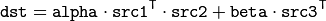# Arithm Operations on Matrices¶

## cuda::gemm¶

Performs generalized matrix multiplication.

C++: void cuda::gemm(InputArray src1, InputArray src2, double alpha, InputArray src3, double beta, OutputArray dst, int flags=0, Stream& stream=Stream::Null())
Parameters: src1 – First multiplied input matrix that should have CV_32FC1 , CV_64FC1 , CV_32FC2 , or CV_64FC2 type. src2 – Second multiplied input matrix of the same type as src1 . alpha – Weight of the matrix product. src3 – Third optional delta matrix added to the matrix product. It should have the same type as src1 and src2 . beta – Weight of src3 . dst – Destination matrix. It has the proper size and the same type as input matrices. flags – Operation flags: GEMM_1_T transpose src1 GEMM_2_T transpose src2 GEMM_3_T transpose src3 stream – Stream for the asynchronous version.

The function performs generalized matrix multiplication similar to the gemm functions in BLAS level 3. For example, gemm(src1, src2, alpha, src3, beta, dst, GEMM_1_T + GEMM_3_T) corresponds toNote

Transposition operation doesn’t support CV_64FC2 input type.

gemm()

## cuda::mulSpectrums¶

Performs a per-element multiplication of two Fourier spectrums.

C++: void cuda::mulSpectrums(InputArray src1, InputArray src2, OutputArray dst, int flags, bool conjB=false, Stream& stream=Stream::Null())
Parameters: src1 – First spectrum. src2 – Second spectrum with the same size and type as a . dst – Destination spectrum. flags – Mock parameter used for CPU/CUDA interfaces similarity. conjB – Optional flag to specify if the second spectrum needs to be conjugated before the multiplication. stream – Stream for the asynchronous version.

Only full (not packed) CV_32FC2 complex spectrums in the interleaved format are supported for now.

mulSpectrums()

## cuda::mulAndScaleSpectrums¶

Performs a per-element multiplication of two Fourier spectrums and scales the result.

C++: void cuda::mulAndScaleSpectrums(InputArray src1, InputArray src2, OutputArray dst, int flags, float scale, bool conjB=false, Stream& stream=Stream::Null())
Parameters: src1 – First spectrum. src2 – Second spectrum with the same size and type as a . dst – Destination spectrum. flags – Mock parameter used for CPU/CUDA interfaces similarity. scale – Scale constant. conjB – Optional flag to specify if the second spectrum needs to be conjugated before the multiplication.

Only full (not packed) CV_32FC2 complex spectrums in the interleaved format are supported for now.

mulSpectrums()

## cuda::dft¶

Performs a forward or inverse discrete Fourier transform (1D or 2D) of the floating point matrix.

C++: void cuda::dft(InputArray src, OutputArray dst, Size dft_size, int flags=0, Stream& stream=Stream::Null())
Parameters: src – Source matrix (real or complex). dst – Destination matrix (real or complex). dft_size – Size of a discrete Fourier transform. flags – Optional flags: DFT_ROWS transforms each individual row of the source matrix. DFT_SCALE scales the result: divide it by the number of elements in the transform (obtained from dft_size ). DFT_INVERSE inverts DFT. Use for complex-complex cases (real-complex and complex-real cases are always forward and inverse, respectively). DFT_REAL_OUTPUT specifies the output as real. The source matrix is the result of real-complex transform, so the destination matrix must be real.

Use to handle real matrices ( CV32FC1 ) and complex matrices in the interleaved format ( CV32FC2 ).

The source matrix should be continuous, otherwise reallocation and data copying is performed. The function chooses an operation mode depending on the flags, size, and channel count of the source matrix:

• If the source matrix is complex and the output is not specified as real, the destination matrix is complex and has the dft_size size and CV_32FC2 type. The destination matrix contains a full result of the DFT (forward or inverse).
• If the source matrix is complex and the output is specified as real, the function assumes that its input is the result of the forward transform (see the next item). The destination matrix has the dft_size size and CV_32FC1 type. It contains the result of the inverse DFT.
• If the source matrix is real (its type is CV_32FC1 ), forward DFT is performed. The result of the DFT is packed into complex ( CV_32FC2 ) matrix. So, the width of the destination matrix is dft_size.width / 2 + 1 . But if the source is a single column, the height is reduced instead of the width.

dft()

## cuda::Convolution¶

class cuda::Convolution : public Algorithm

Base class for convolution (or cross-correlation) operator.

class CV_EXPORTS Convolution : public Algorithm
{
public:
virtual void convolve(InputArray image, InputArray templ, OutputArray result, bool ccorr = false, Stream& stream = Stream::Null()) = 0;
};


## cuda::Convolution::convolve¶

Computes a convolution (or cross-correlation) of two images.

C++: void cuda::Convolution::convolve(InputArray image, InputArray templ, OutputArray result, bool ccorr=false, Stream& stream=Stream::Null())
Parameters: image – Source image. Only CV_32FC1 images are supported for now. templ – Template image. The size is not greater than the image size. The type is the same as image . result – Result image. If image is W x H and templ is w x h, then result must be W-w+1 x H-h+1. ccorr – Flags to evaluate cross-correlation instead of convolution. stream – Stream for the asynchronous version.

## cuda::createConvolution¶

Creates implementation for cuda::Convolution .

C++: Ptr<Convolution> createConvolution(Size user_block_size=Size())
Parameters: user_block_size – Block size. If you leave default value Size(0,0) then automatic estimation of block size will be used (which is optimized for speed). By varying user_block_size you can reduce memory requirements at the cost of speed.